# explain Two sides and an angle are given. Determine whether the given information results in one...

explain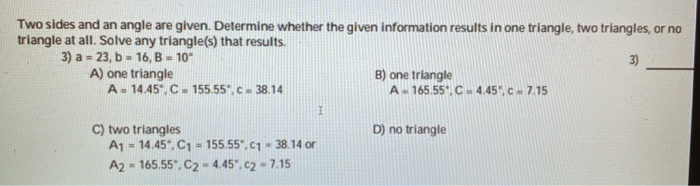Two sides and an angle are given. Determine whether the given information results in one triangle, two triangles, or no triangle at all. Solve any triangle(s) that results. 3) a = 23, b = 16, B = 10" A) one triangle B) one triangle A - 14.45%, C - 155.55°C-38.14 A - 165.55'. C4.45.C -7.15 D) no triangle C) two triangles A1 - 14.45". C1 - 155.55*.c1 - 38.14 or A2 - 165.55. C2 - 4.45*c2-7.15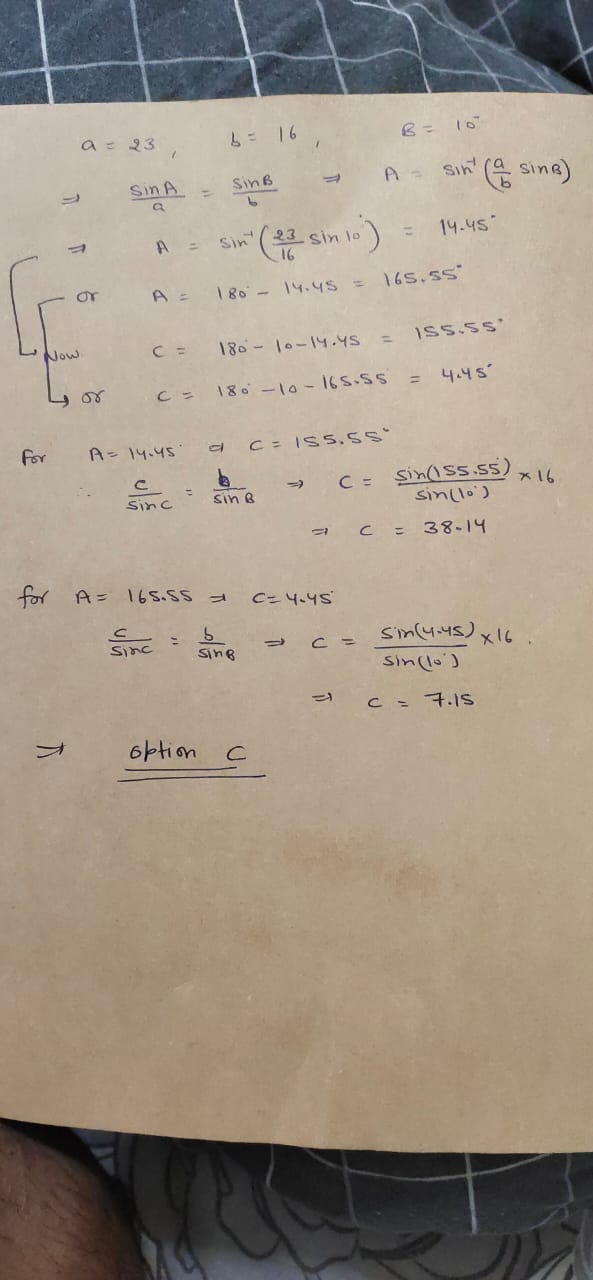#### Earn Coin

Coins can be redeemed for fabulous gifts.

Similar Homework Help Questions
• ### please explain why the right answer is C Two sides and an angle are given. Determine...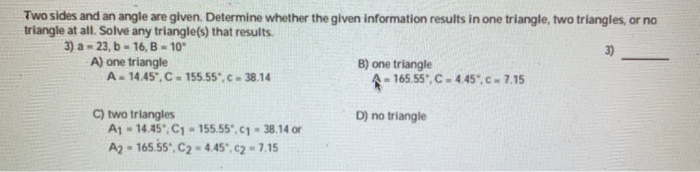please explain why the right answer is C Two sides and an angle are given. Determine whether the given information results in one triangle, two triangles, or no triangle at all. Solve any triangle(s) that results. 3) a = 23,6 - 16,B - 10" A) one triangle B) one triangle A - 14.45.C - 155.55.c.38.14 A-165.55.C.4.45.c.7.15 D) no triangle C) two triangles A1 - 14.45%, C1 - 155.55.1 - 38.14 or A2 - 165.55*, C2 = 4.45*, C2 - 7.15

• ### Two sides and an angle are given. Determine whether the given information results in one triangle,...

Two sides and an angle are given. Determine whether the given information results in one triangle, two triangles, or no triangle at all. Solve any triangle(s) that results. b=5 c=9 B=85degree Please step by step!

• ### Two sides and an angle are given below. Determine whether the given information results in one...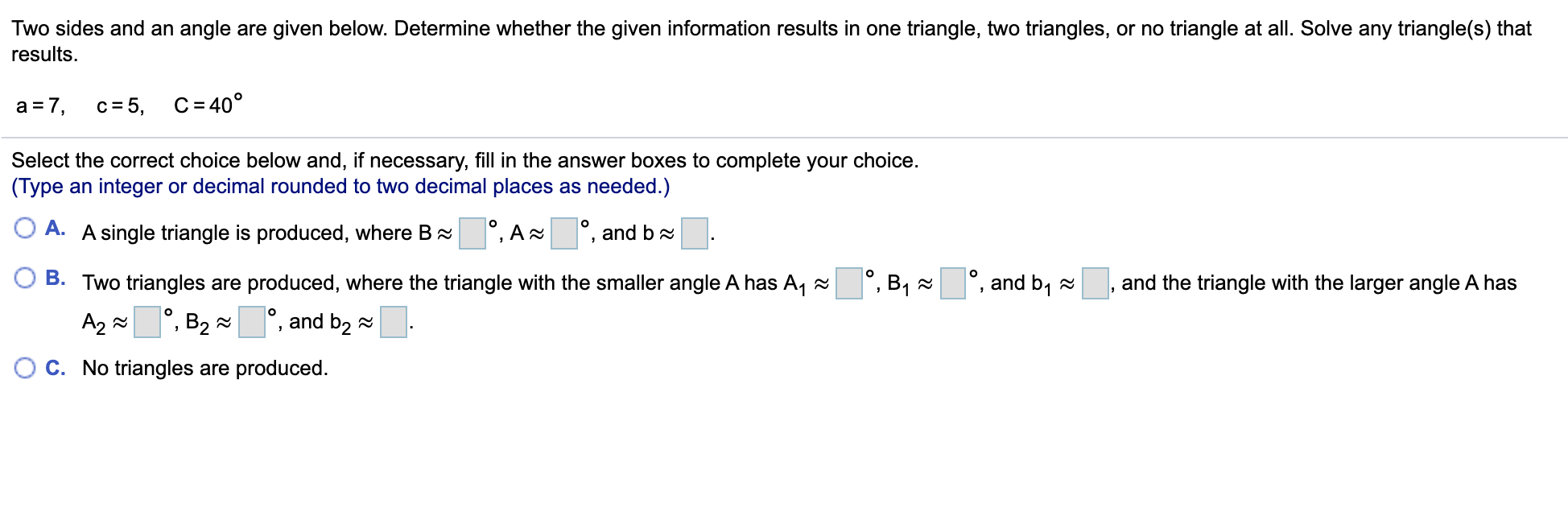Two sides and an angle are given below. Determine whether the given information results in one triangle, two triangles, or no triangle at all. Solve any triangle(s) that results. a = 7, c=5, C = 40° Select the correct choice below and, if necessary, fill in the answer boxes to complete your choice. (Type an integer or decimal rounded to two decimal places as needed.) º, By a °, and by ~ , and the triangle with the larger angle...

• ### 8.2.35 Two sides and an angle are given below. Determine whether the given information results in...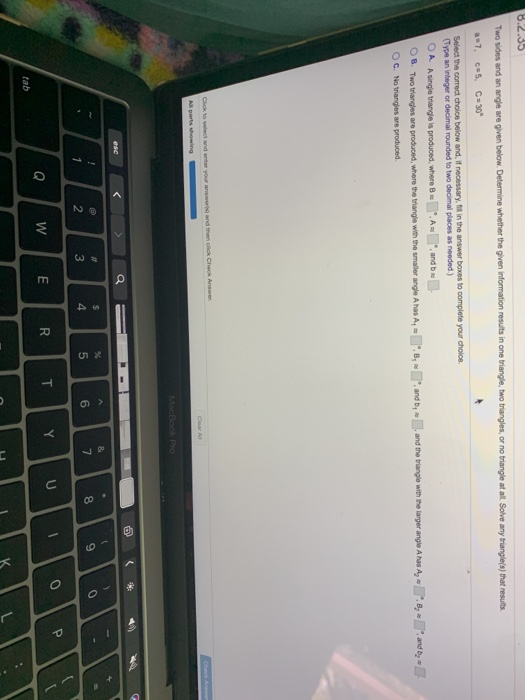8.2.35 Two sides and an angle are given below. Determine whether the given information results in one triangle, two triangles, or no triangle at all Solve any triangle(s) that results a 7. c=5. C=30° Select the correct choice below and, if necessary, in in the answer boxes to complete your choice. Type an integer or decimal rounded to two decimal places as needed.) OA Asinge triangle is produced, where B .AN . and be OB Two Wanges are produced, where...

• ### To. D In Exercises 17-32, two sides and an angle (SSAI given. Determine whether the given measure...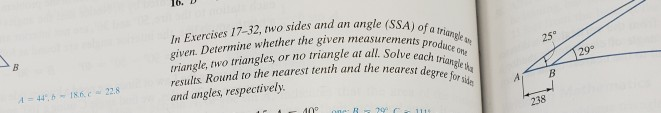To. D In Exercises 17-32, two sides and an angle (SSAI given. Determine whether the given measurements triangle, two triangles, or no triangle at all. Solve results Round to the nearest tenth and the neat a and angles, respectively of a triangle 259 produce one 29 each trian rest degree for side gle ka 238 To. D In Exercises 17-32, two sides and an angle (SSAI given. Determine whether the given measurements triangle, two triangles, or no triangle at all....

• ### 6. Two sides and an angle of a triangle are given. Determine whether the measureinents produce...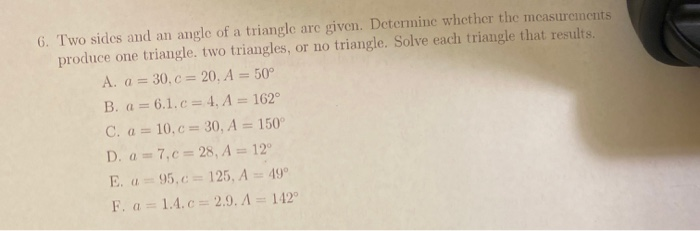6. Two sides and an angle of a triangle are given. Determine whether the measureinents produce one triangle. two triangles, or no triangle. Solve each triangle that results. A. a = 30.C = 20. A = 50° Ba= = 6.1.C = 4. A = 162° C. a = 10,0 = 30, A = 150° D. a = 7,0 = 28, A = 12° E. a = 95.c = 125, A = 49° F. a = 1.4.C = 2.9. A =...

• ### Two sides and an angle (SSA) of a triangle are given. Determine whether the given measurements produce one triangle, two triangles, or no triangle at all Solve each trianglo that results A:53° 15...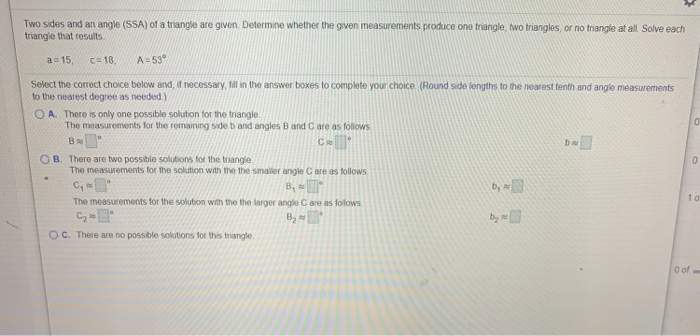Two sides and an angle (SSA) of a triangle are given. Determine whether the given measurements produce one triangle, two triangles, or no triangle at all Solve each trianglo that results A:53° 15, c:18, cpoitn rer t Select the correct choice below and, if necessary ill in the answer boxes to complete your choice (Round side lengths to the nearest tenth and angle measurements to the nearest degree as needed.) O A. There is only one possible solution for the...

• ### 6. Two sides and an angle of a triangle are given. Determine whether the measurements produce...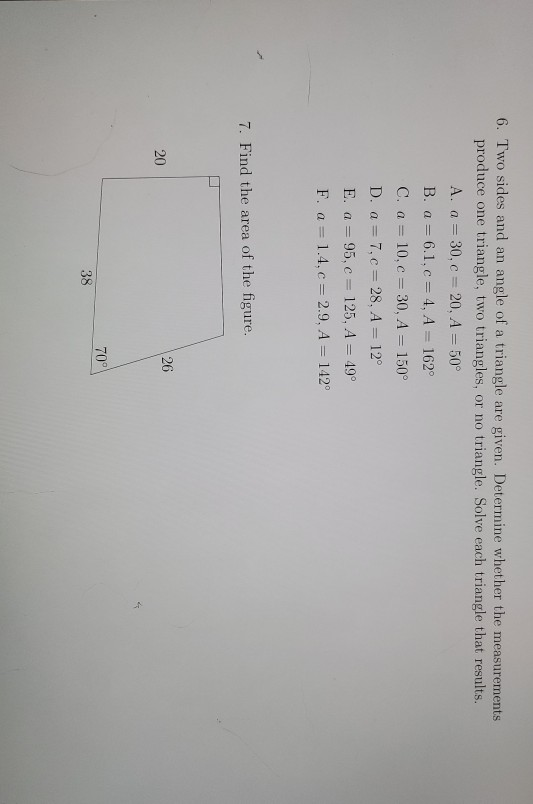6. Two sides and an angle of a triangle are given. Determine whether the measurements produce one triangle, two triangles, or no triangle. Solve each triangle that results. A. a = 30,C=20, A = 50° B. a = 6.1.c = 4, A = 162° C. a = 10.c = 30, A = 150° D. a = 7, c = 28, A = 12° E. a = 95, C = 125, A = 49° F. a = 1.4.c = 2.9, A...

• ### Q6 4 pts) In each of the following, two sides and an angle of a triangle...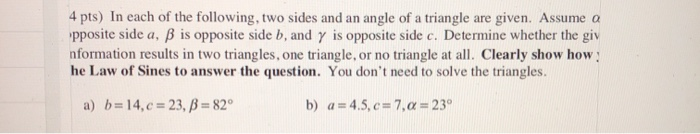Q6 4 pts) In each of the following, two sides and an angle of a triangle are given. Assume a 'pposite side a, ß is opposite side b, and y is opposite side c. Determine whether the giv nformation results in two triangles, one triangle, or no triangle at all. Clearly show how he Law of Sines to answer the question. You don't need to solve the triangles. a) b= 14,c = 23, B = 82° b) a = 4.5,c=7,a...

• ### 2. Determine whether the information results in one triangle, two triangles, or no triangle. Solve any...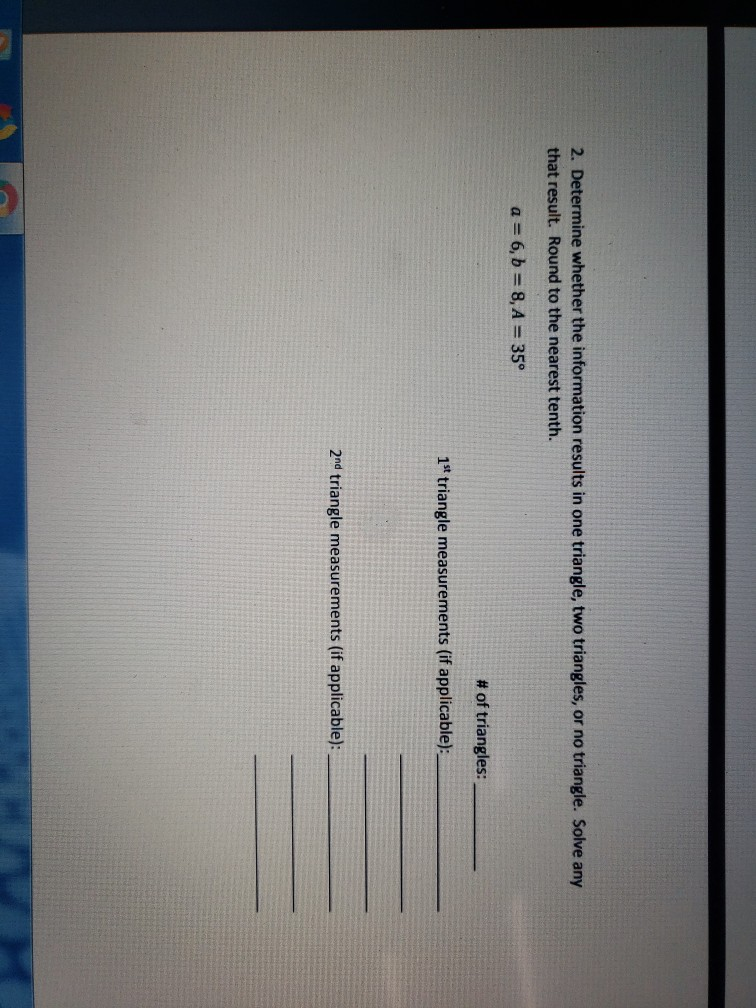2. Determine whether the information results in one triangle, two triangles, or no triangle. Solve any that result. Round to the nearest tenth. a = 6, b = 8, A = 35° # of triangles: 1s triangle measurements (if applicable): 2nd triangle measurements (if applicable):